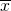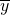Discrete Random Variables

# Discrete Distribution (Lucky Dice Experiment)

Discrete Distribution (Lucky Dice Experiment)

Class Time:

Names:

Student Learning Outcomes
• The student will compare empirical data and a theoretical distribution to determine if a Tet gambling game fits a discrete distribution.
• The student will demonstrate an understanding of long-term probabilities.
Supplies
• one “Lucky Dice” game or three regular dice

Procedure
Round answers to relative frequency and probability problems to four decimal places.

1. The experimental procedure is to bet on one object. Then, roll three Lucky Dice and count the number of matches. The number of matches will decide your profit.
2. What is the theoretical probability of one die matching the object?
3. Choose one object to place a bet on. Roll the three Lucky Dice. Count the number of matches.
4. Let X = number of matches. Theoretically, X ~ B(______,______)
5. Let Y = profit per game.

Organize the DataIn (Figure), fill in the y value that corresponds to each x value. Next, record the number of matches picked for your class. Then, calculate the relative frequency.

1. Complete the table.
x y Frequency Relative Frequency
0
1
2
3
2. Calculate the following:
1.= _______
2. sx = ________
3.= _______
4. sy = _______
3. Explain whatrepresents.
4. Explain whatrepresents.
5. Based upon the experiment:
1. What was the average profit per game?
2. Did this represent an average win or loss per game?
3. How do you know? Answer in complete sentences.
6. Construct a histogram of the empirical data.Theoretical DistributionBuild the theoretical PDF chart for x and y based on the distribution from the Procedure section.

1. x y P(x) = P(y)
0
1
2
3
2. Calculate the following:
1. μx = _______
2. σx = _______
3. μx = _______
3. Explain what μx represents.
4. Explain what μy represents.
5. Based upon theory:
1. What was the expected profit per game?
2. Did the expected profit represent an average win or loss per game?
3. How do you know? Answer in complete sentences.
6. Construct a histogram of the theoretical distribution.Use the Data

Note

RF = relative frequency

Use the data from the Theoretical Distribution section to calculate the following answers. Round your answers to four decimal places.

1. P(x = 3) = _________________
2. P(0 < x < 3) = _________________
3. P(x ≥ 2) = _________________

Use the data from the Organize the Data section to calculate the following answers. Round your answers to four decimal places.

1. RF(x = 3) = _________________
2. RF(0 < x < 3) = _________________
3. RF(x ≥ 2) = _________________

Discussion QuestionFor questions 1 and 2, consider the graphs, the probabilities, the relative frequencies, the means, and the standard deviations.

1. Knowing that data vary, describe three similarities between the graphs and distributions of the theoretical and empirical distributions. Use complete sentences.
2. Describe the three most significant differences between the graphs or distributions of the theoretical and empirical distributions.
3. Thinking about your answers to questions 1 and 2, does it appear that the data fit the theoretical distribution? In complete sentences, explain why or why not.
4. Suppose that the experiment had been repeated 500 times. Would you expect (Figure) or (Figure) to change, and how would it change? Why? Why wouldn’t the other table change?